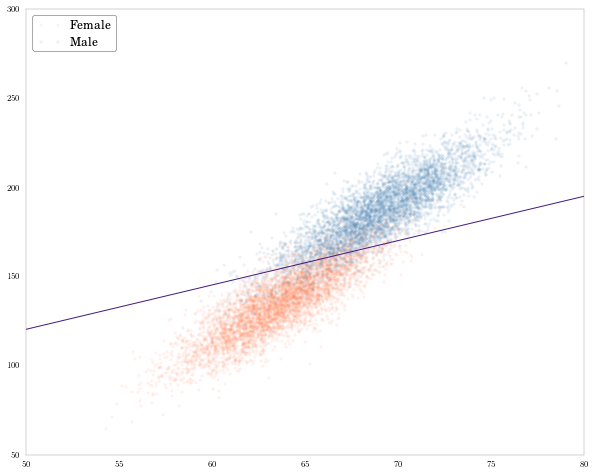In :
''''
-------------------------------------------------------------------------------
Filename   : ch2.ipynb
Date       : 2012-04-30
Author     : C. Vogel
Purpose    : Replicate analysis of height and weight data in Chapter 2 of
: _Machine Learning for Hackers_.
Input Data : 01_heights_weights_genders.tsv is available at the book's github
: repository at https://github.com/johnmyleswhite/ML_for_Hackers.git
Libraries  : Numpy 1.6.1, Matplotlib 1.1.0, Pandas 0.7.3, scipy 0.10.0,
: statsmodels 0.4.0
-------------------------------------------------------------------------------

This notebook is a Python port of the R code in Chapter 2 of _Machine Learning
for Hackers_ by D. Conway and J.M. White.

Running the notebook will produce 9 PNG figures and save them to the working
directory.

The height/weight dataset CSV file should be located in a /data/ subfolder of
the working directory.

For a detailed description of the analysis and the process of porting it
to Python, see: slendrmeans.wordpress.com/will-it-python.
'''

Out:
&quot;&apos;\n-------------------------------------------------------------------------------\nFilename   : ch2.ipynb\nDate       : 2012-04-30\nAuthor     : C. Vogel\nPurpose    : Replicate analysis of height and weight data in Chapter 2 of \n           : _Machine Learning for Hackers_.\nInput Data : 01_heights_weights_genders.tsv is available at the book&apos;s github \n           : repository at https://github.com/johnmyleswhite/ML_for_Hackers.git\nLibraries  : Numpy 1.6.1, Matplotlib 1.1.0, Pandas 0.7.3, scipy 0.10.0, \n           : statsmodels 0.4.0\n-------------------------------------------------------------------------------\n\nThis notebook is a Python port of the R code in Chapter 2 of _Machine Learning\nfor Hackers_ by D. Conway and J.M. White.\n\nRunning the notebook will produce 9 PNG figures and save them to the working \ndirectory.\n\nThe height/weight dataset CSV file should be located in a /data/ subfolder of \nthe working directory. \n\nFor a detailed description of the analysis and the process of porting it\nto Python, see: slendrmeans.wordpress.com/will-it-python.\n&quot;
In :
import numpy as np
from pandas import *
import matplotlib.pyplot as plt
import os
import statsmodels.api as sm
from statsmodels.nonparametric.kde import KDE
from statsmodels.nonparametric import lowess
from statsmodels.api import GLM, Logit

In :
# Numeric Summaries
# p. 37

In :
# Import the height and weights data

In :
# Assign the heights column to its own series, and describe it.
heights = heights_weights['Height']
heights.describe()

Out:
count    10000.000000
mean        66.367560
std          3.847528
min         54.263133
25%         63.505620
50%         66.318070
75%         69.174262
max         78.998742
In :
# Means, medians, and modes (p. 38)

In :
def my_mean(x):
return float(np.sum(x)) / len(x)

In :
def my_median(x):
'''
Compute the median of a series x.
'''

# Get a sorted copy of the values in the series (need to call values
# otherwise integer indexing messes things up.)
sorted_x = np.sort(x.values)
if len(x) % 2 == 0:
indices = [0.5 * len(x) - 1, 0.5 * len(x)]
return np.mean(sorted_x[indices])
else:
# Ceil(x) - 1 = Floor(x), but this is to make clear that the -1 is to
# account for 0-based counting.
index = ceil(0.5 * len(x)) - 1
return sorted_x.ix[index]

In :
# Check my_mean and my_median against built-ins

In :
my_mean(heights) - heights.mean()

Out:
0.0
In :
my_median(heights) - heights.median()

Out:
0.0
In :
# Quantiles (p. 40)

In :
heights.min(), heights.max()

Out:
(54.263133325097101, 78.998742346389605)
In :
# Range = max - min. Note: np.ptp(heights.values) will do the same thing.
# HT Nathaniel Smith

In :
def my_range(s):
'''
Difference between the max and min of an array or Series
'''
return s.max() - s.min()

my_range(heights)

Out:
24.735609021292504
In :
# Similarly, pandas doesn't seem to provide multiple quantiles.
# But (1) the standard ones are available via .describe() and
# (2) creating one is simple.

In :
# To get a single quantile
heights.quantile(.5)

Out:
66.31807008178464
In :
# Function to get arbitrary quantiles of a series.
def my_quantiles(s, prob = (0.0, 0.25, 0.5, 1.0)):
'''
Calculate quantiles of a series.

Parameters:
-----------
s : a pandas Series
prob : a tuple (or other iterable) of probabilities at
which to compute quantiles. Must be an iterable,
even for a single probability (e.g. prob = (0.50)
not prob = 0.50).

Returns:
--------
A pandas series with the probabilities as an index.
'''
q = [s.quantile(p) for p in prob]
return Series(q, index = prob)

# With the default prob argument
my_quantiles(heights)

Out:
0.00    54.263133
0.25    63.505620
0.50    66.318070
1.00    78.998742
In :
# With a specific prob argument - here deciles
my_quantiles(heights, prob = arange(0, 1.1, 0.1))

Out:
0.0    54.263133
0.1    61.412701
0.2    62.859007
0.3    64.072407
0.4    65.194221
0.5    66.318070
0.6    67.435374
0.7    68.558072
0.8    69.811620
0.9    71.472149
1.0    78.998742
In :
 # Standard deviation and variances

In :
def my_var(x):
return np.sum((x - x.mean())**2) / (len(x) - 1)

In :
my_var(heights) - heights.var()

Out:
-2.2854607095723622e-11
In :
def my_sd(x):
return np.sqrt(my_var(x))

In :
my_sd(heights) - heights.std()

Out:
-2.9700686354772188e-12
In :
# Exploratory Data Visualization (p. 44)

In :
# Histograms

In :
# 1-inch bins
bins1 = np.arange(heights.min(), heights.max(), 1.)
heights.hist(bins = bins1, fc = 'steelblue')
plt.savefig('height_hist_bins1.png')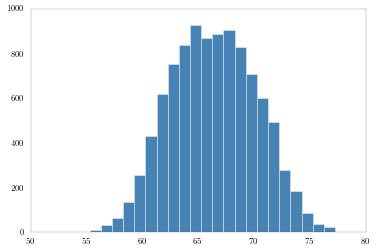In :
# 5-inch bins
bins5 = np.arange(heights.min(), heights.max(), 5.)
heights.hist(bins = bins5, fc = 'steelblue')
plt.savefig('height_hist_bins5.png')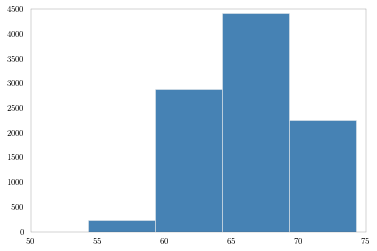In :
# 0.001-inch bins
bins001 = np.arange(heights.min(), heights.max(), .001)
heights.hist(bins = bins001, fc = 'steelblue')
plt.savefig('height_hist_bins001.png')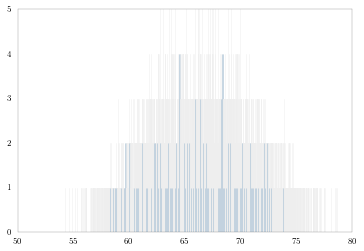In :
# Kernel density estimators, from scipy.stats.

In :
# Create a KDE ojbect
heights_kde = KDE(heights)
# Use fit() to estimate the densities. Default is gaussian kernel
# using fft. This will provide a "density" attribute.
heights_kde.fit()

In :
# Plot the density of the heights
# Sort inside the plotting so the lines connect nicely.
fig = plt.figure()
plt.plot(heights_kde.support, heights_kde.density)
plt.savefig('heights_density.png')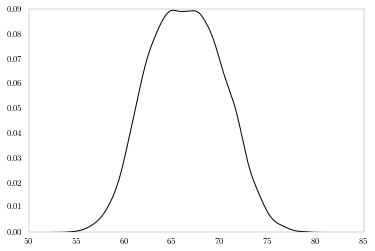In :
# Pull out male and female heights as arrays over which to compute densities

heights_m = heights[heights_weights['Gender'] == 'Male'].values
heights_f = heights[heights_weights['Gender'] == 'Female'].values
heights_m_kde = KDE(heights_m)
heights_f_kde = KDE(heights_f)
heights_m_kde.fit()
heights_f_kde.fit()

fig = plt.figure()
plt.plot(heights_m_kde.support, heights_m_kde.density, label = 'Male')
plt.plot(heights_f_kde.support, heights_f_kde.density, label = 'Female')
plt.legend()
plt.savefig('height_density_bysex.png')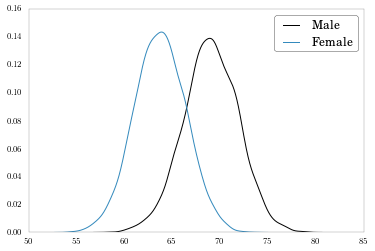In :
# Do the same thing with weights.
weights_m = heights_weights[heights_weights['Gender'] == 'Male']['Weight'].values
weights_f = heights_weights[heights_weights['Gender'] == 'Female']['Weight'].values
weights_m_kde = KDE(weights_m)
weights_f_kde = KDE(weights_f)
weights_m_kde.fit()
weights_f_kde.fit()

fig = plt.figure()
plt.plot(weights_m_kde.support, weights_f_kde.density, label = 'Male')
plt.plot(weights_f_kde.support, weights_f_kde.density, label = 'Female')
plt.legend()
plt.savefig('weight_density_bysex.png')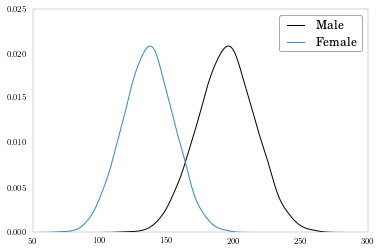In :
# Subplot weight density by sex.
fig, axes = plt.subplots(nrows = 2, ncols = 1, sharex = True, figsize = (9, 6))
axes.plot(weights_f_kde.support, weights_f_kde.density, label = 'Female')
axes.xaxis.tick_top()
axes.legend()
axes.plot(weights_m_kde.support, weights_f_kde.density, label = 'Male')
axes.legend()
plt.savefig('weight_density_bysex_subplot.png')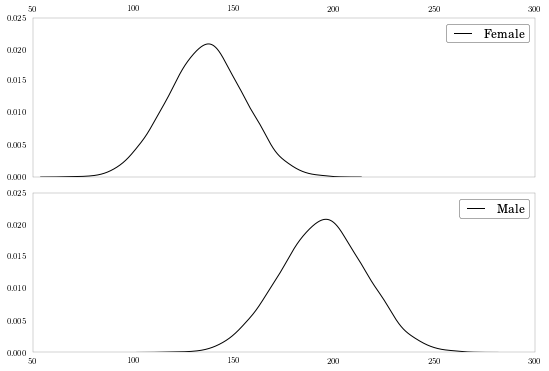In :
# Scatter plot. Pull weight (both sexes) out as a separate array first, like
# we did with height above.

In :
weights = heights_weights['Weight']
plt.plot(heights, weights, '.k', mew = 0, alpha = .1)
plt.savefig('height_weight_scatter.png')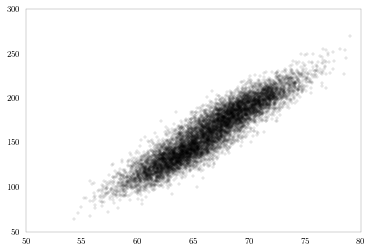In :
# Lowess smoothing - this seems to be new functionality not yet in docs (as of 0.40, April 2012).

In :
lowess_line = lowess.lowess(weights, heights)

In :
plt.figure(figsize = (13, 9))
plt.plot(heights, weights, '.', mfc = 'steelblue', mew=0, alpha = .25)
plt.plot(lowess_line[:,0], lowess_line[:, 1],  '-', color = '#461B7E', label = "Lowess fit")
plt.legend(loc = "upper left")
plt.savefig('height_weight_lowess.png')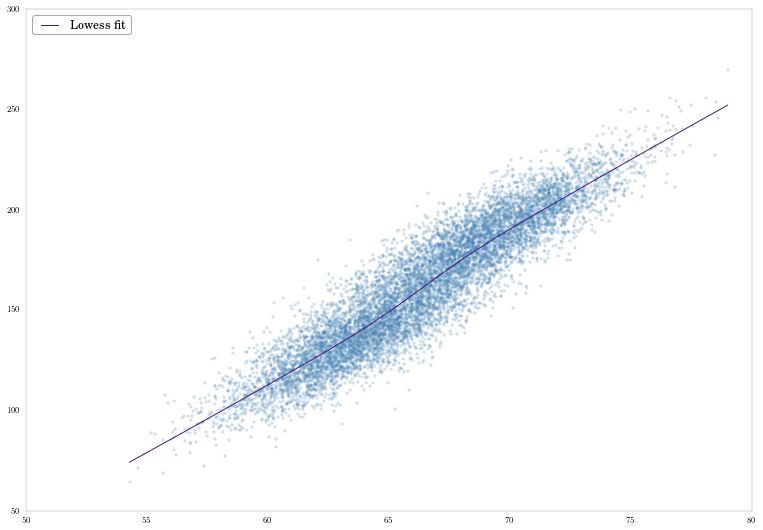In :
# Logistic regression of sex on height and weight
# Sex is coded in the binary variable male.

In :
# LHS binary variable
male = (heights_weights['Gender'] == 'Male') * 1

In :
# Matrix of predictor variables: hieght and weight from data frame
# into an Nx2 array.
hw_exog = heights_weights[['Height', 'Weight']].values

In :
# Logit model 1: Using GLM and the Binomial Family w/ the Logit Link
# Note I have to add constants to the exog matrix. The prepend = True
# argument prevents a warning about future change to the default argument.
logit_model.fit().summary()

Out:
Dep. Variable: No. Observations: Gender 10000 GLM 9997 Binomial 2 logit 1.0 IRLS -2091.3 Tue, 08 May 2012 4182.6 14:24:49 9.72e+03 8
coef std err t P>|t| [95.0% Conf. Int.] 0.6925 1.328 0.521 0.602 -1.911 3.296 -0.4926 0.029 -17.013 0.000 -0.549 -0.436 0.1983 0.005 38.663 0.000 0.188 0.208
In :
# Get the coefficient parameters.
logit_pars = logit_model.fit().params

In :
# Logit model 2: Using the Logit function.
logit_model2 = Logit(male, sm.add_constant(hw_exog, prepend = True))
logit_model2.fit().summary()

Optimization terminated successfully.
Current function value: 2091.297971
Iterations 8
Out:
Dep. Variable: No. Observations: Gender 10000 Logit 9997 MLE 2 Tue, 08 May 2012 0.6983 14:24:51 -2091.3 True -6931.5 0
coef std err z P>|z| [95.0% Conf. Int.] 0.6925 1.328 0.521 0.602 -1.911 3.296 -0.4926 0.029 -17.013 0.000 -0.549 -0.436 0.1983 0.005 38.663 0.000 0.188 0.208
In :
# Get the coefficient parameters
logit_pars2 = logit_model2.fit().params

Optimization terminated successfully.
Current function value: 2091.297971
Iterations 8
In :
# Compare the two methods again. They give the same parameters.
DataFrame({'GLM' : logit_pars, 'Logit' : logit_pars2})

Out:
GLM Logit
const 0.692543 0.692543
x1 -0.492620 -0.492620
x2 0.198340 0.198340
In :
# Draw a separating line in the [height, weight]-space.
# The line will separate the space into predicted-male
# and predicted-female regions.

In :
# Get the intercept and slope of the line based on the logit coefficients
intercept = -logit_pars['const'] / logit_pars['x2']
slope =  -logit_pars['x1'] / logit_pars['x2']

In :
# Plot the data and the separating line
# Color code male and female points.
fig = plt.figure(figsize = (10, 8))
plt.plot(heights_f, weights_f, '.', label = 'Female', mew = 0, mfc='coral', alpha = .1)
plt.plot(heights_m, weights_m, '.', label = 'Male', mew = 0, mfc='steelblue', alpha = .1)
plt.plot(array([50, 80]), intercept + slope * array([50, 80]), '-', color = '#461B7E')
plt.legend(loc='upper left')
plt.savefig('height_weight_class.png')